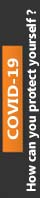2019-01-25T10:56:26+00:00 Assignments

# Design a greedy algorithm using pseudocode that solves this optimization problem of transferring files to disk while minimizing unused storage.

This paper concentrates on the primary theme of Design a greedy algorithm using pseudocode that solves this optimization problem of transferring files to disk while minimizing unused storage. in which you have to explain and evaluate its intricate aspects in detail. In addition to this, this paper has been reviewed and purchased by most of the students hence; it has been rated 4.8 points on the scale of 5 points. Besides, the price of this paper starts from £ 40. For more details and full access to the paper, please refer to the site.

# Designing a greedy algorithm

Question: Designing a greedy algorithm

Part 1

Design a greedy algorithm using pseudocode that solves this optimization problem of transferring files to disk while minimizing unused storage. The inputs to this algorithm are the number of files n, corresponding sizes (in MBs) s1, … sn, m the number of disks, and corresponding storages amounts t1, …, tm. The algorithm should return an array map[i] which contains the disk index of which the ith media file should be stored.

Part 2

Discuss the optimality of your algorithm. Is it guaranteed to return an optimal result?

What is the Big-O time complexity of this algorithm in terms of m and n? Justify your answer.

Part 3

If you were to solve this problem using a brute force or exhaustive search method, what would be the time complexity? Justify your response.

When designing a greedy algorithm, you always want to grab the nearest solution instead of searching for the most optimal one.

Thus, first start out by heap-sorting:
++ the files in descending order of size
++ the disks in descending order of storage amount
However, preserve the original indices of the files and disks.

Now, you have sorted binary heaps:
++ files[] of size n, and for i = 1 to n the sizes of each files[i] in s[i]
++ disks[] of size m, and for i = 1 to m the storage amounts of each disks[i] in t[i]

I’m going to assume that files cannot be stored across disks: either a file can be on a disk or it cannot, no partial storage.

Start with the files (the largest file) and disks (the largest disk, i.e. i = 1 to n, j = 1 to m
if disk[j].capacity < files[i].size:
next disk to check is disks[j].right
else
store the file, reduce the disk storage amount by the file size, move to the next file

“store the file” means map[the original index of files[i]] = the original index of disks[j]. So, you need to preserve the original indices.

Optimality: If we wanted to get an optimal solution, we’d recursively store each possible mapping of file to disk and calculate the optimal solution from that recursion, then implement that optimal solution. That’s dynamic programming. However, we are being greedy: we want the shortest and quickest checking and while that will be correct, it may not necessarily produce the optimal solution.

Complexity: O(nlogn + mlogm + nlogm)
We sort each array. Then, we iterate over all files, and for each file iterate down a tree of disks: height of tree is log(number of nodes).

Brute force: no sorting, no tree, only two nested loops. For each file, check every disk. O(nm).

0% Plagiarism Guaranteed & Custom Written, Tailored to your instructions

International House, 12 Constance Street, London, United Kingdom,
E16 2DQ

## STILL NOT CONVINCED?

We've produced some samples of what you can expect from our Academic Writing Service - these are created by our writers to show you the kind of high-quality work you'll receive. Take a look for yourself!FLAT 25% OFF ON EVERY ORDER.Use "FLAT25" as your promo code during checkout